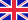•Topological graph theory

Autor: Source: Wikipedia

Source: Wikipedia. Pages: 86. Chapters: Albertson conjecture, Betti number, Book embedding, Combinatorial map, Conway's thrackle conjecture, Covering space, Crossing number (graph theory), Cycle double cover, Dessin d'enfant, Dual graph, Euler characteristic,... Viac o knihe

Na objednávku, dodanie 2-4 týždne

21.59 €

bežná cena: 25.40 €

O knihe

Source: Wikipedia. Pages: 86. Chapters: Albertson conjecture, Betti number, Book embedding, Combinatorial map, Conway's thrackle conjecture, Covering space, Crossing number (graph theory), Cycle double cover, Dessin d'enfant, Dual graph, Euler characteristic, Fáry's theorem, Fork (topology), Fraysseix-Rosenstiehl's planarity criterion, Generalized map, Genus (mathematics), Graph drawing, Graph embedding, Heawood conjecture, Heawood number, Linkless embedding, Regular map (graph theory), Road coloring problem, Rotation system, String graph, Toroidal graph, Water, gas, and electricity. Excerpt: 284 article summaries including: Topological Graph Theory from Japan . Problems in topological graph theory . Computational Results in Topological Graph Theory . PROBLEMS IN TOPOLOGICAL GRAPH THEORY : QUESTIONS I CAN'T ANSWER . 11 A computational approach to Conway's thrackle conjecture . 16 The road coloring problem . 17 Towards The Albertson Conjecture . 21 Small oriented cycle double cover of graphs . 22 On The Cycle Double Cover Problem . 24 The Generalized Road Coloring Problem and periodic digraphs . Graph drawing . 27 On Simultaneous Graph Embedding . Distributed Social Graph Embedding . 31 A Constructive Proof of the Cycle Double Cover Conjecture . Euler characteristic of Fredholm quasicomplexes . Mapping degree and Euler characteristic . Graph Drawing Contest Report . Graph-Drawing Contest Report . Graph-Drawing Contest Report . The Graph Drawing Server . Graph-Drawing Contest Report . Validating Graph Drawing Aesthetics . Graph-Drawing Contest Report . Graph Drawing Contest Report . Graph-Drawing Contest Report . Graph Drawing Contest Report . Online Hierarchical Graph Drawing . Graph-Drawing Contest Report . Graph Drawing Contest Report . Three-Dimensional Graph Drawing . Graph-Drawing Contest Report . Graph Drawing Contest Report . Graph-Drawing Contest Report . Validating Graph Drawing Aesthetics . Graph-Drawing Contest Report . The Graph Drawing Server . Online Hierarchical Graph Drawing . Graph-Drawing Contest Report . Three-Dimensional Graph Drawing . Graph-Drawing Contest Report . Graph Drawing Contest Report . Graph-Drawing Contest Report . Layered graph drawing . Graph Drawing Contest Report . Planar Graph Drawing . Constrained Graph Drawing . Spectral graph drawing . Layered graph drawing . Principles of Graph drawing . Skinable graph drawing . Covering space (ruang covering) . Stability and the first Betti number . The fragment assembly string graph . 104 The Euler characteristic of a Hecke algebra . 105 The Euler characteristic of a category . 106 Validity of the expected Euler characteristic heuristic . 107 Unusual formulae for the Euler characteristic . 108 On Euler Characteristic of equivariant sheaves . 109 Local holomorphic Euler characteristic and instanton decay . 110 Euler characteristic and quadrilaterals of normal surfaces . 111 The Euler characteristic of a polyhedral product . 112 Constant curvature hyperspheres and the Euler Characteristic . 115 Degree formula for the Euler characteristic . 116 Displaceability and the mean Euler characteristic . 117 Varieties with vanishing holomorphic Euler characteristic . The Euler characteristic of a category . Euler characteristic structure and weight homology . Cohomogeneity one manifolds with positive Euler characteristic . Displaceability and the mean Euler characteristic . Validity of the expected Euler characteristic heur...

• Vydavateľstvo: Books LLC, Reference Series
• Rok vydania: 2012
• Formát: Paperback
• Rozmer: 246 x 189 mm
• Jazyk: Anglický jazyk
• ISBN: 9781156622889

Generuje redakčný systém BUXUS CMS spoločnosti ui42.